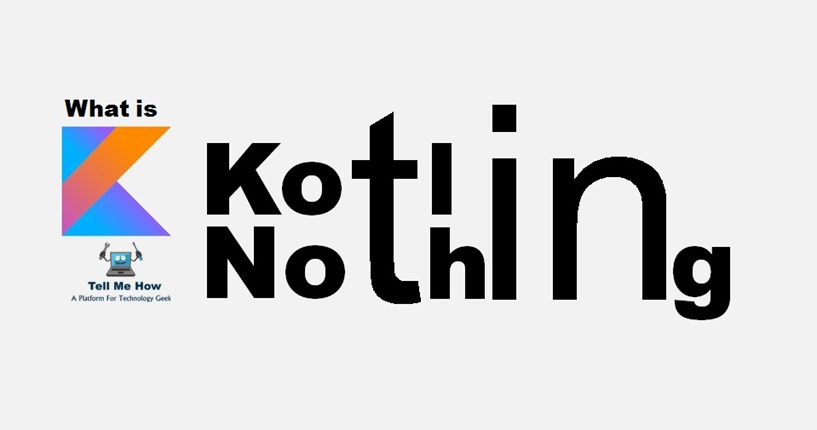## What is Nothing Type in Kotlin?A colleague approached me a while ago and asked me a question about Kotlin. What was this “Nothing type”, and what was it used for? I did not really have a good answer for him at the time. Since then, I’ve found my answer.

### Nothing Type is the Bottom Type

In mathematics, bottom (often denoted as _|_) represents the absolute minimum value something can hold.

Nothing type has no instances. You can use Nothing to represent “a value that never exists”: for example, if a function has the return type of Nothing, it means that it never returns (always throws an exception).

Previously shared Kotlin post:

When we’re talking about types, we can think of `Nothing` as being the absolute bottom (leaf) object of any type hierarchy.

What we can see in this diagram (figure 1) is that `Nothing` is the opposite of `Any`. While every object you will work with in Kotlin implicitly extends `Any`, so to does `Nothing` implicity extend any object that exists. This makes `Nothing` the ‘dual’ or opposite of `Any`.

So, why is this useful?

### Covariant Types (an Example)

We can use `Nothing` along with covariant (producer) types to help make our code more concise and expressive. The following is an example of the `Nothing` type in action. It is of an `Either` monad, which can exclusively represent one of two types (`L` or `R`), and provides methods for mapping over the values it wraps, including a right-biased `map` and `flatMap` (by convention) and a very useful `either` method.

```// Composes 2 functions
fun <A, B, C> ((A) -> B).c(f: (B) -> C): (A) -> C = {
f(this(it))
}

sealed class Either<out L, out R> {
data class Left<out L>(val a: L) : Either<L, Nothing>()
data class Right<out R>(val b: R) : Either<Nothing, R>()

val isRight: Boolean
get() = this is Right<R>

val isLeft: Boolean
get() = this is Left<L>
}

fun <L> left(a: L) = Either.Left(a)
fun <R> right(b: R) = Either.Right(b)

fun <T, L, R> Either<L, R>.flatMap(fn: (R) -> Either<L, T>): Either<L, T> =
when (this) {
is Either.Left -> Either.Left(a)
is Either.Right -> fn(b)
}

fun <T, L, R> Either<L, R>.map(fn: (R) -> (T)): Either<L, T> = this.flatMap(fn.c(::right))

fun <T, L, R> Either<L, R>.either(fnL: (L) -> T, fnR: (R) -> T): T =
when (this) {
is Either.Left -> fnL(a)
is Either.Right -> fnR(b)
}```

Covariance (marking our parameters as `out`) allows us to utilize nothing in the `R` and `L` positions of the `Left` and `Right` declarations of the `Either`monad implemented here (respectively). Our functions all typecheck and are happy. For example, something like:

`val e = right(42).flatMap(r -> left(Exception())`

Will happily type-check and compile. We can even rigidly express the type of `e` and it’s still happy as can be, all thanks to `Nothing` and covariance:

```val e: Either<Int, Exception> = right(42)
.flatMap { left(Exception() }```

You might have noticed that extension methods are being used here for `map``flatMap`, and so on. This is due to the Covariant typing mentioned above, which produces type-check errors when trying to pass a lambda utilizing the generic types. Extension methods are a quick and easy way around this issue.

### So what did we actually get?

What we end up with is a better, more concise way to express our types. Nothing almost acts like a type hole, similar to utilizing `_` in lambda arguments. We can use it in places where we really just don’t care about the type of something. In our case, if we’ve got a `Right`, we don’t really need to know anything about the error, because it doesn’t exist. On the other hand, if we have a `Left`, knowledge of the error is necessary, but not so much for the normal value.

Hopefully this sheds some light on why `Nothing` exists and how it can be leveraged.#### By Tell Me How

It is a technology blog and admin has excellent experience in programming from 5+ year. You can contact us at ceo.tellmehow@gmail.com

#### 12 Best Hacking Apps for Android